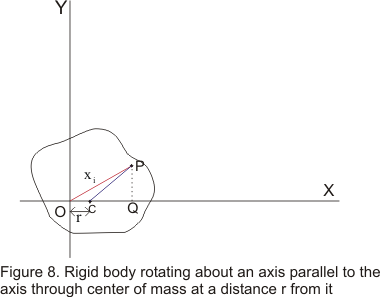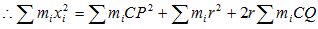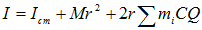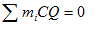# Theorems of Moment of Inertia|Parallel axis theorem

## (6) Theorems of Moment of Inertia

• There are two general theorems which proved themselves to be of great importance on moment of inertia
• These enable us to determine moment of inertia of a body about an axis if moment of inertia of body about some other axis is known
• The theorems are Parallel Axis theorem and Perpendicular axis theorem

ii) Parallel axis theorem
• This theorem relates the moment of inertia about an axis through the Center of mass of a body about a second parallel axis
• Let Icm be the moment of inertia about an axis through center of mass of the body and I be that about a parallel axis at a distance r from C as shown below in the figureThen according to parallel axis theorem
I=Icm+Mr2 where M is the total mass of the body
• Consider a point P of the body of mass mi at a distance xi from O• From point P drop a perpendicular PQ on to the OC and join PC.So that
OP2=CP2+ OC2+ 2OC.CQ ( From geometry) and miOP2=miCP2+ miOC2+ 2miOC.CQ• Since the body always balances about an axis passing through center of mass, so algebraic sum of the moment of the weight of individual particles about center of mass must be zero. Herewhich is the algebraic sum of such moments about C and therefore eq as g is constant
Thus we have I=Icm + Mr2 ---(17)

Question 1. Moment of inertia of a uniform rod of length L and mass M about an axis passing through L/4 from one end and perpendicular to its length
a. 7ML2/36
b.7ML2/48
c. 11ML2/48
d.ML2/12

Solution 1

Using parallel axis theorem
I=Icm+Mx2 where x is the distance of the axis of the rotation from the CM of the rod
So x=L/2-L/4=L/4 Also Icm=ML2 /12

So I=ML2 /12+ML2 /16=7ML2 /48